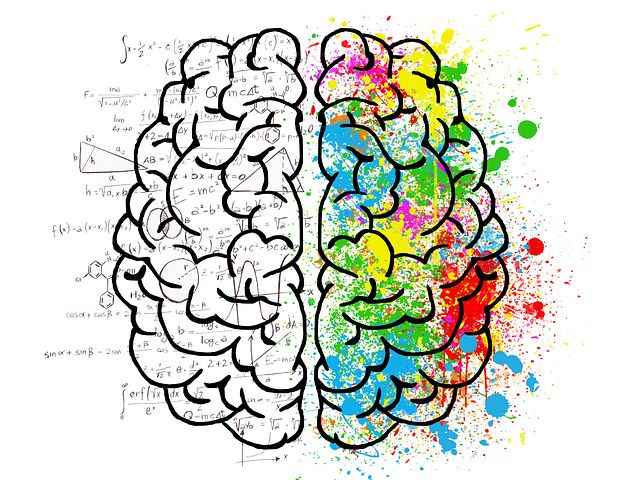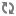## using task transfer to solve data driven problems

in this article we would see how we could use a trained model, which is called VGG-16 which is a deep convolutional neural network (CNN) and was used to classify about 1,000,000 images from the ImageNet dataset which has 15,000,000 images from 1,000 classes, and how we are going to task transfer it to solve a different problem. the different problem is classifying The flowers dataset which collected in Volkani institute: The data includes 473 cropped images of flowers and non-flowers, with corresponding labels. The original images from which the flower and non-flower rectangles were cropped from are not given, due to their large size. we will use the first 300 cropped images for training. Test on the remaining 173. You will have to resize the images to 224X224X3, which is the input shape of the pre-trained CNNs on imagenet. Notice that in some network implementations the input shape might be 227X227X3. we would only train the last 3 layers of the network, which are all fully connected layers.

for solving the problem we would use Keras package with tensorflow backend. of course you could use any other package for this problem, like pytorch, and with other backend like Tehano. so first let's import the required packages.

```from keras import Input
from keras.applications import VGG16
from keras.models import Model
from keras.applications.imagenet_utils import preprocess_input
from keras.layers import Flatten, Dropout, Dense, TimeDistributed, MaxPooling2D
from keras import backend as backend
from keras import optimizers
from keras import regularizers
from keras.applications.vgg16 import preprocess_input
import tensorflow as tf
from tensorflow.python.keras.callbacks import TensorBoard
from sklearn.metrics import precision_recall_curve, accuracy_score, log_loss
from sklearn.utils.fixes import signature
import multiprocessing
import scipy.io
import pandas as pd
import numpy as np
import matplotlib.pyplot as plt
import cv2
import time
import pickle
from datetime import datetime
import os
import random
from numba import cuda
import json```

now we need to configure the model, that means we need to import the VGG model, and to determine that we need to train only the last 3 layers.

```def configure_model(dropout, l1, l2, size):
"""
configuring the model architecture
:param dropout: the dropout chance to use
:param dropout: the dropout chance
:param l1: the regularization weight for l1 for weight decay
:param l2: the regularization weight for l2 for weight decay
:param size: the width and height of a picture
:return: a configured model
"""
image_input = Input(shape=(size, size, 3))
model = VGG16(weights='imagenet', include_top=True, input_tensor=image_input)
last_layer = model.get_layer('block5_pool').output
x = Flatten(name='flatten')(last_layer)
x = Dropout(dropout)(x)
x = Dense(512, activation='relu', name='fc1')(x)
x = Dropout(dropout)(x)
x = Dense(512, activation='relu', name='fc2')(x)
x = Dropout(dropout)(x)
out = Dense(params["num_classes"], activation='sigmoid', name='output',
kernel_regularizer=regularizers.l1_l2(l1=l1, l2=l2))(x)
model = Model(image_input, out)

# freeze all the layers except the dense layers
for layer in model.layers[:-3]:
layer.trainable = False

return model```

as you can see in the for loop which is in line 25 we accomplished this goal, we are changing the parameter of trainable to false for all the layers except the last 3. and we replaced the last 3 layers to ones with relu activation function and 512 neurons in each hidden layer. our loss function is sigmoid because we only have 2 classes compared to the original softmax loss function which was more suitable for the problem of 1,000 classes. now all that is left is to run the model with some hyper parameters to fine tune, we are not going to cover that because i assume that you have a basic understanding of deep learning hyper parameters. so all is left now is to run the model.

```if __name__ == '__main__':

start_all_over = False
levels = ["basic", "improved"]
configure(gpu_ind=False)
params = set_params()
start_time = time.time()
resized_images, Y = prepare_data(params)
X_train, X_test, y_train, y_test = train_test_split_first(resized_images, Y, params["first_n_split"])
if start_all_over:
for level in levels:
if level == "improved":
X_train, y_train = augmentation(X_train, y_train, params)
best_model, batch_size = train_grid_search(X_train, y_train, params, level)
report(best_model, X_test, y_test, batch_size, level, params["first_n_split"])
else:
X_train, y_train = augmentation(X_train, y_train, params)
best_model, batch_size = get_best_model(X_train, y_train)
report(best_model, X_test, y_test, batch_size, "improved", params["first_n_split"])
print("the total time is: {} minutes".format((time.time() - start_time) / 60))
plt.show()```

the code snippet above is the main function that we run for solving the problem, we solve 2 types of solution one is basic and the other one is more advanced because it uses more techniques like augmentation which is a process of generating more images as rotations of existing ones. the main function also find the best hyper parameters and comparing them by generating an precision-recall curve. the train_grid_search function is very straight forward and was created as for loops for reasons of using this code in self implemented way, this is not the best practice of course, but it will help you debug the code more easily.

the results we got where pretty good after taking into consideration the hyper parameters space we searched and the amount of samples we had: